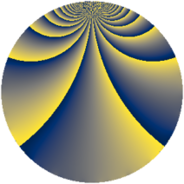# Properties

 Label 1045.2.bcLevel $1045$ Weight $2$ Character orbit 1045.bc Rep. character $\chi_{1045}(229,\cdot)$ Character field $\Q(\zeta_{10})$ Dimension $432$ Sturm bound $240$

# Related objects

## Defining parameters

 Level: $$N$$ $$=$$ $$1045 = 5 \cdot 11 \cdot 19$$ Weight: $$k$$ $$=$$ $$2$$ Character orbit: $$[\chi]$$ $$=$$ 1045.bc (of order $$10$$ and degree $$4$$) Character conductor: $$\operatorname{cond}(\chi)$$ $$=$$ $$55$$ Character field: $$\Q(\zeta_{10})$$ Sturm bound: $$240$$

## Dimensions

The following table gives the dimensions of various subspaces of $$M_{2}(1045, [\chi])$$.

Total New Old
Modular forms 496 432 64
Cusp forms 464 432 32
Eisenstein series 32 0 32

## Trace form

 $$432 q + 108 q^{4} + 8 q^{6} + 92 q^{9} + O(q^{10})$$ $$432 q + 108 q^{4} + 8 q^{6} + 92 q^{9} - 24 q^{10} - 2 q^{11} + 8 q^{14} + 6 q^{15} - 164 q^{16} - 8 q^{19} - 8 q^{21} - 8 q^{24} + 16 q^{25} - 24 q^{26} + 8 q^{29} + 24 q^{30} - 28 q^{31} - 16 q^{34} - 56 q^{35} - 100 q^{36} - 96 q^{39} - 20 q^{40} + 24 q^{41} - 40 q^{44} - 34 q^{45} + 96 q^{46} + 106 q^{49} + 64 q^{51} + 120 q^{54} + 11 q^{55} - 8 q^{56} + 12 q^{59} - 24 q^{60} + 42 q^{61} + 268 q^{64} + 24 q^{65} + 72 q^{66} - 4 q^{69} + 108 q^{70} - 68 q^{71} - 164 q^{74} - 10 q^{75} - 80 q^{76} - 48 q^{79} - 76 q^{80} - 128 q^{81} + 8 q^{84} - 35 q^{85} - 144 q^{86} - 104 q^{89} - 260 q^{90} - 96 q^{94} + 3 q^{95} + 324 q^{96} + 198 q^{99} + O(q^{100})$$

## Decomposition of $$S_{2}^{\mathrm{new}}(1045, [\chi])$$ into newform subspaces

The newforms in this space have not yet been added to the LMFDB.

## Decomposition of $$S_{2}^{\mathrm{old}}(1045, [\chi])$$ into lower level spaces

$$S_{2}^{\mathrm{old}}(1045, [\chi]) \cong$$ $$S_{2}^{\mathrm{new}}(55, [\chi])$$$$^{\oplus 2}$$